# fitgeotrans

(Not recommended) Fit geometric transformation to control point pairs

`fitgeotrans` is not recommended. Use the `fitgeotform2d` function instead. For more information, see Compatibility Considerations.

## Syntax

``tform = fitgeotrans(movingPoints,fixedPoints,tformType)``
``tform = fitgeotrans(movingPoints,fixedPoints,"polynomial",degree)``
``tform = fitgeotrans(movingPoints,fixedPoints,"pwl")``
``tform = fitgeotrans(movingPoints,fixedPoints,"lwm",n)``

## Description

example

````tform = fitgeotrans(movingPoints,fixedPoints,tformType)` fits a linear geometric transformation of type `tformType` to control point pairs `movingPoints` and `fixedPoints`.```
````tform = fitgeotrans(movingPoints,fixedPoints,"polynomial",degree)` fits a `PolynomialTransformation2D` object to control point pairs `movingPoints` and `fixedPoints`. Specify the degree of the polynomial transformation, which can be 2, 3, or 4.```
````tform = fitgeotrans(movingPoints,fixedPoints,"pwl")` fits a `PiecewiseLinearTransformation2D` object to control point pairs `movingPoints` and `fixedPoints`. This transformation creates a Delaunay triangulation of the fixed control points, and maps moving control points to the corresponding fixed control points. A different affine transformation maps control points in each local region. The mapping is continuous across the control points, but is not continuously differentiable.```
````tform = fitgeotrans(movingPoints,fixedPoints,"lwm",n)` fits a `LocalWeightedMeanTransformation2D` object to control point pairs `movingPoints` and `fixedPoints`. The local weighted mean transformation creates a mapping, by inferring a polynomial at each control point using neighboring control points. The mapping at any location depends on a weighted average of these polynomials. The `n` closest points are used to infer a second degree polynomial transformation for each control point pair.```

## Examples

collapse all

Create a checkerboard image and rotate it to create a misaligned image.

```I = checkerboard(40); J = imrotate(I,30); imshowpair(I,J,"montage")```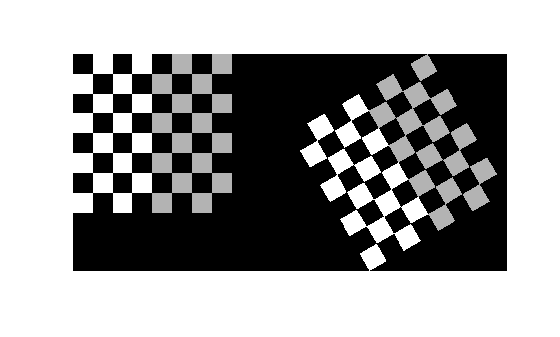Define some matching control points on the fixed image (the checkerboard) and moving image (the rotated checkerboard). You can define points interactively using the Control Point Selection tool.

```fixedPoints = [41 41; 281 161]; movingPoints = [56 175; 324 160];```

Create an affine geometric transformation that can be used to align the two images.

`tform = fitgeotform2d(movingPoints,fixedPoints,"similarity");`

Use the `tform` estimate to resample the rotated image to register it with the fixed image. The regions of color (green and magenta) in the false color overlay image indicate error in the registration. This error comes from a lack of precise correspondence in the control points.

```Jregistered = imwarp(J,tform,OutputView=imref2d(size(I))); imshowpair(I,Jregistered)```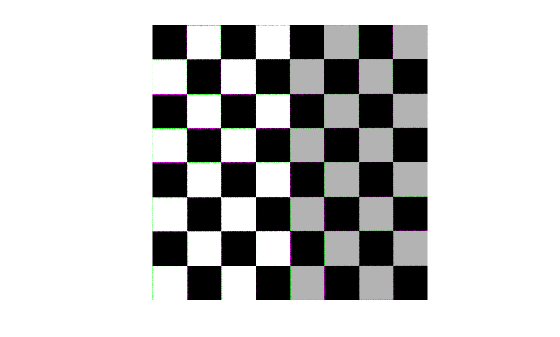## Input Arguments

collapse all

Control points in the moving image, specified as an m-by-2 matrix. Each row specifies the (x, y) coordinate of a control point.

Example: `movingPoints = [11 11; 41 71];`

Data Types: `double` | `single`

Control points in the fixed image, specified as an m-by-2 matrix. Each row specifies the (x, y) coordinate of a control point.

Example: `fixedPoints = [14 44; 70 81];`

Data Types: `double` | `single`

Type of linear transformation, specified as `"nonreflectivesimilarity"`, `"similarity"`, `"affine"`, or `"projective"`. For more information, see Transformation Types.

Data Types: `char` | `string`

Degree of the polynomial, specified as the integer `2`, `3`, or `4`.

Data Types: `single` | `double` | `int8` | `int16` | `int32` | `int64` | `uint8` | `uint16` | `uint32` | `uint64`

Number of points to use in local weighted mean calculation, specified as a positive integer. `n` can be as small as 6, but making `n` small risks generating ill-conditioned polynomials.

Data Types: `single` | `double` | `int8` | `int16` | `int32` | `int64` | `uint8` | `uint16` | `uint32` | `uint64`

## Output Arguments

collapse all

Transformation, returned as a geometric transformation object. The type of object depends on the transformation type.

Transformation Type

Geometric Transformation Object
`"nonreflectivesimilarity"``affine2d`
`"similarity"``affine2d`
`"affine"``affine2d`
`"projective"``projective2d`
`"polynomial"``PolynomialTransformation2D`
`"pwl"``PiecewiseLinearTransformation2D`
`"lwm"``LocalWeightedMeanTransformation2D`

collapse all

### Transformation Types

The table lists all the transformation types supported by `fitgeotrans` in order of complexity.

Transformation Type

DescriptionMinimum Number of Control Point PairsExample
`"nonreflectivesimilarity"`Use this transformation when shapes in the moving image are unchanged, but the image is distorted by some combination of translation, rotation, and scaling. Straight lines remain straight, and parallel lines are still parallel. 2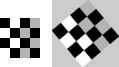`"similarity"`Same as `"nonreflectivesimilarity"` with the addition of optional reflection.3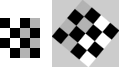`"affine"`Use this transformation when shapes in the moving image exhibit shearing. Straight lines remain straight, and parallel lines remain parallel, but rectangles become parallelograms.3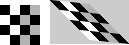`"projective"`Use this transformation when the scene appears tilted. Straight lines remain straight, but parallel lines converge toward a vanishing point.4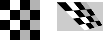`"polynomial"`Use this transformation when objects in the image are curved. The higher the order of the polynomial, the better the fit, but the result can contain more curves than the fixed image.

6 (order 2)

10 (order 3)

15 (order 4)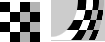`"pwl"`Use this transformation (piecewise linear) when parts of the image appear distorted differently.4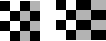`"lwm"`Use this transformation (local weighted mean) when the distortion varies locally and piecewise linear is not sufficient. 6 (12 recommended)Goshtasby, Ardeshir. “Piecewise Linear Mapping Functions for Image Registration.” Pattern Recognition 19, no. 6 (January 1986): 459–66. https://doi.org/10.1016/0031-3203(86)90044-0.

 Goshtasby, Ardeshir. “Image Registration by Local Approximation Methods.” Image and Vision Computing 6, no. 4 (November 1988): 255–61. https://doi.org/10.1016/0262-8856(88)90016-9.